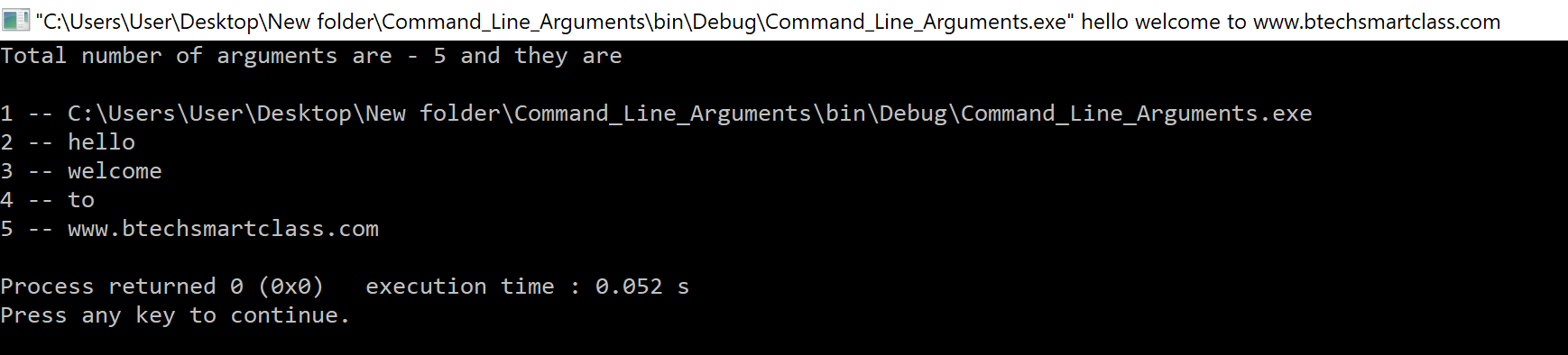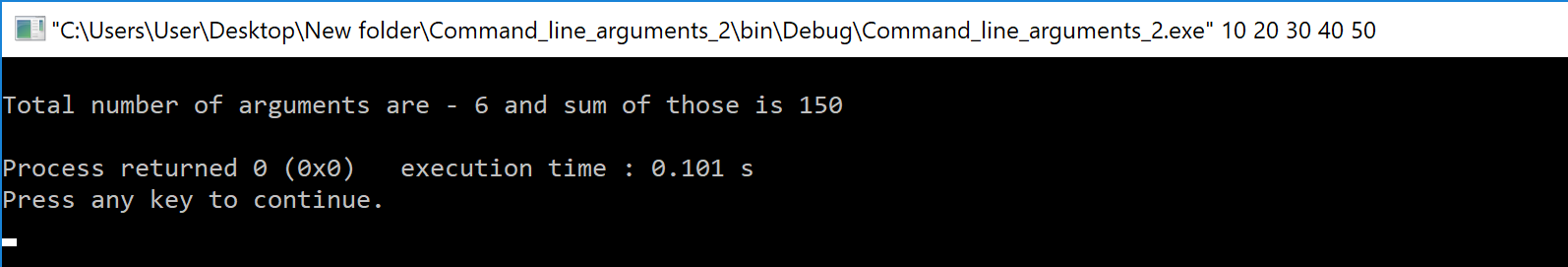The perfect place for easy learning...

×

# Command Line Arguments in C

In C programming language, command line arguments are the data values that are passed from command line to our program. Using command line arguments we can control the program execution from the outside of the program. Generally, all the command line arguments are handled by the main() method. Generally, the command line arguments can be understood as follows...

Command line arguments are the parameters passing to main() method from the command line.

When command line arguments are passed main() method receives them with the help of two formal parameters and they are,

• int argc
• char *argv[ ]

int argc - It is an integer argument used to store the count of command line arguments are passed from the command line.

char *argv[ ] - It is a character pointer array used to store the actual values of command line arguments are passed from the command line.

Importent Points to be Remembered
The command line arguments are supperated with SPACE.
Always the first command line argument is file path.
Only string values can be passed as command line arguments.
All the command line arguments are stored in a character pointer array called argv[ ].
Total count of command line arguments including file path argument is stored in a integer parameter called argc.

Consider the following example program...

# Example Program to illustrate command line arguments in C.

``````#include<stdio.h>
#include<conio.h>

int main(int argc, char *argv[]){

int i;
clrscr() ;

if(argc == 1){
return 0;
}
else{
printf("Total number of arguments are - %d and they are\n\n", argc);
for(i=0; i<argc ; i++){
printf("%d -- %s \n", i+1, argv[i]);
}
return 0;
}
}```
```

When execute the above program by passing "Hello welcome to www.btechsmartclass.com" as command line arguments it produce the following output.In the above example program we are passing 4 string arguments (Hello, welcome, to and www.btechsmartclass.com) but the default first argument is a file path. So, the total count of command line arguments is 5.

Whenever we want to pass numerical values as command line arguments, they are passed as string values only and we need to convert them into numerical values in the program. Consider the following program that calculates the sum of all command line arguments and displays the result.

# Example Program to display sum of all command line arguments in C.

``````#include<stdio.h>
#include<conio.h>
#include<stdlib.h>

int main(int argc, char *argv[]){

int i, n, sum = 0;
clrscr() ;

if(argc == 1){
return 0;
}
else{
printf("Total number of arguments are - %d and sum of those is ", argc);
for(i=0; i<argc ; i++){
n = atoi(argv[i]);
sum += n;
}
printf("%d\n", sum);
return 0;
}
}```
```

When execute the above program by passing "10 20 30 40 50" as command line arguments it produce the following output.In the above example program we are passing 5 string arguments (10, 20, 30 40 and 50). They are converted into integer values by using atoi() method which is available in stdlib.h header file.